# Lines toolbar

#####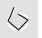Convert

Change a curve to polyline segments.

#####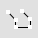CurveThroughPt

Draw a polyline (degree 1 curve) through points.

#####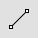Line

Draw a single line segment.

#####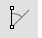Line, Angled

Draw one angled line segment.

#####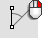Line, Angled, BothSides

Draw one angled line segment from its midpoint.

#####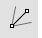Line, Bisector

Draw one line segment at a bisecting angle.

#####Line, Bisector, BothSides

Draw one line segment at a bisecting angle from its midpoint.

#####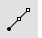Line, BothSides

Draw one line segment from its midpoint.

#####Line, FourPoint

Draw one line segment by four points.

#####Line, FourPoint, BothSides

Draw one line segment by four points from its midpoint.

#####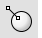Line, Normal

Draw a line segment normal to a surface.

#####Line, Normal, BothSides

Draw a line segment normal to a surface from its midpoint.

#####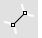Line, Perpendicular, 2Curves

Draw one line segment perpendicular to two curves.

#####Line, Perpendicular From

Draw a line segment perpendicular from a curve.

#####Line, Perpendicular To

Draw a line segment perpendicular to a curve from its midpoint.

#####Line, Tangent, 2Curves

Draw one line segment tangent to two curves.

#####Line, Tangent From

Draw a line segment tangent from a curve.

#####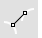Line, Tangent, Perpendicular

Draw one line segment tangent and perpendicular to curves.

#####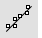LineThroughPt

Fit a line through any combination of points, control points, and point-cloud objects.

#####Line, Vertical

Draw a line segment perpendicular to the construction plane.

#####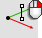Line, Vertical, BothSides

Draw a line segment perpendicular to the construction plane from its midpoint.

#####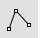Polyline

Draw a multi-segment polyline with options for line and arc segments, tracking line helpers, and close.